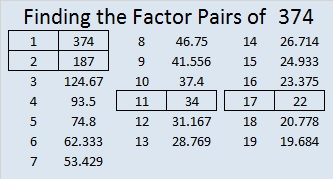# 374 and Level 5

374 is even so it is a composite number. Its factoring information is below the puzzle.Print the puzzles or type the factors on this excel file:12 Factors 2015-01-26

• 374 is a composite number.
• Prime factorization: 374 = 2 x 11 x 17
• The exponents in the prime factorization are 1, 1, and 1. Adding one to each and multiplying we get (1 + 1)(1 + 1)(1 + 1) = 2 x 2 x 2 = 8. Therefore 374 has exactly 8 factors.
• Factors of 374: 1, 2, 11, 17, 22, 34, 187, 374
• Factor pairs: 374 = 1 x 374, 2 x 187, 11 x 34, or 17 x 22
• 374 has no square factors that allow its square root to be simplified. √374 ≈ 19.339